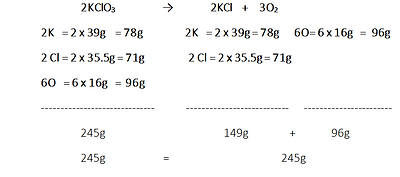top of pageExample Videos

## Physiology Lab

Click to expand

Muscarinic receptors would be found in which location(s) in the diagram

A. Heart Rate

B. TPR

C. EDV

D. SV

E. MAP

Increasing the viscosity of blood would directly affect:

A. TPR

B. CO

C. MAP

D. EDV

E. SV

The change in the radius of a vessel, raised to the fourth power can affect blood flow according to which principle Poiseuille’s Law.  EDV changes directly impact what factor?

A. CO

B. TPR

C. MAP

D. SV

In the case of a hemorrhage, which of the following events might occur?

A. Vasoconstriction of vessels due to release        Acetylcholine (Ach)

B. Decrease Heart Rate

C. Increase release Norepinephrine (NE) release

D. Elevated End Systolic Volume

Select all the structures in the flow diagram where Norepinephrine would act on alpha-1 receptor by clicking of structures in chart.

A. Venous return

B. Cardiac Output

C. TPR

D. SV

Muscarinic receptors are stimulated by:

A. Acetylcholine****

B. Norepinephrine

C. Nicotine

D. Gaba

E. Dopamine

## Chemistry Lab

Click to expand

Stoichiometry Exercise

In this laboratory exercise, you will need to understand how to use the masses of the elements/compounds to show the Law of Conservation of Mass in the decomposition reaction of a KCLO3.

The Stoichiometry laboratory exercise deals with the decomposition of potassium chlorate using manganese dioxide as a catalyst. The purpose of this activity is to conduct an experiment dealing with the decomposition of potassium chlorate in order to:

• To illustrate how the Law of Conservation of Mass is obeyed in a balanced chemical reaction.

• Identify the reactants and products in a balanced chemical reaction.

• Illustrate how to properly setup a chemical decomposition laboratory.

Develop and evaluate the quantitative nature of a chemical experiment using correct stoichiometrical relationships.

Step One: Use the Periodic Table provided to derive mass of each elements.

-Potassium (K) has a mass of 39

-Chlorine (Cl) has a mass of 35.5

-Oxygen (O) has a mass of 16.

Step Two: Add the atomic mass for each compound to demonstrate conservation of mass in the equation.

2KClO3

2K   = 2 x 39g    = 78g

2 Cl = 2 x 35.5g = 71g

6O   = 6 x 16g   =  96g

--------

245g

245g

2KCl    +    3O2

2K   = 2 x 39g = 78g

2 Cl = 2 x 35.5g = 71g

--------

149g

6O=6 x 16g   =  96g

--------

+                       96g

=                                                     245gStep Three: Summary with illustration

In 2KClO     →        2KCl    +    3O we see that atoms and mass is conserved. But the large numbers of  2 :  2 : 3 represents the moles of each reactant, together they represent the molar ratio in the reaction but the moles are not conserved but are critical to stoichiometric calculation as you will see. Therefore the Law of conservation of Mass has been obeyed grams as a unit of mass.

Option to allow students to play with balancing equations

____Na   +   ____H20 → ____NaOH   +   ____H₂

____Zn    +  ____HF  →  ____ZnF2    +      ____H₂

___ Mn(NO) + ___ BeCl₂  ___ Be(NO) + ___ MnClbottom of page